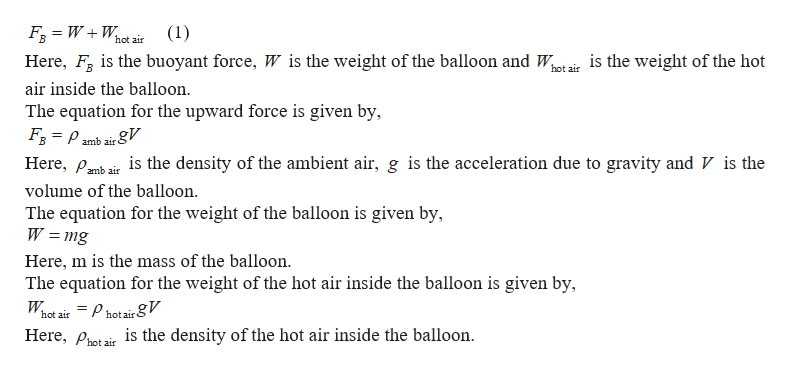# A hot-air balloon consists of a basket hanging beneath a large envelope filled with hot air. A typical hot-air balloon has a total mass of 545 kg, including passengers in its basket, and holds 2.55 x 103 m3 of hot air in its envelope. If the ambient air density is 1.25 kg/m3 , determine the density of hot air inside the envelope when the balloon is neutrally buoyant. Neglect the volume of air displaced by the basket and passengers.

Question
3 views

A hot-air balloon consists of a basket hanging beneath a large envelope filled with hot air. A typical hot-air balloon has a total mass of 545 kg, including passengers in its basket, and holds 2.55 x 103 m3 of hot air in its envelope. If the ambient air density is 1.25 kg/m3 , determine the density of hot air inside the envelope when the balloon is neutrally buoyant. Neglect the volume of air displaced by the basket and passengers.

check_circle

Step 1

The forces acting on the system are the buoyant force acting upward, the weight of the basket acting downward and the weight of the hot air acting...help_outlineImage TranscriptioncloseF = W +W (1) hot air Here, F, is the buoyant force, W is the weight of the balloon and W is the weight of the hot hot air air inside the balloon. The equation for the upward force is given by, F3 = P amb air gV V is the Here, penb sir is the density of the ambient air, g is the acceleration due to gravity and volume of the balloon. The equation for the weight of the balloon is given by, W = mg Here, m is the mass of the balloon. The equation for the weight of the hot air inside the balloon is given by, Whot air = P hotair gV Here, Pretair is the density of the hot air inside the balloon. fullscreen

### Want to see the full answer?

See Solution

#### Want to see this answer and more?

Solutions are written by subject experts who are available 24/7. Questions are typically answered within 1 hour.*

See Solution
*Response times may vary by subject and question.
Tagged in

### Physics